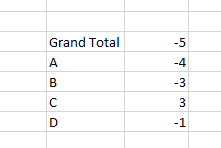# Formulas for top most level

Hello,

I am building a dashboard that has detail as shown below.What I would like to do is create a formula that says if the grand total is less than 0, return 0 else return the grand total.

If I create a formula to give me this as a stand alone measure it 0’s out all the data less than 0 and gives me a total of 3.

The way I was able to get this to work was modifying the Totals Calculation Rules. The issue I have now, however, is that if I want to do math with this measure, it still uses the original sum, not the if statement I used as a specialty total.

Is there a way I can accomplish this?

Thank you,

Did you try something like the following (you’d need to click ‘advanced’ if you don’t want it all on one line).

``````if(SUM(\$measureColumn\$) < 0)
{
return 0;
}
else
{
return SUM(\$measureColumn\$)
}
``````

In general, I find that breaking out the formula into multiple parts and building it up that way helps to work out how to do it.

Hi Patrick,

@david.glickman’s right on this one. Using if else statements would be the way to go in this scenario.

it simplifies things and any math you’d like to perform later would be easy to implement as well.

`Math.Max(0, SUM(\$measureName\$)`